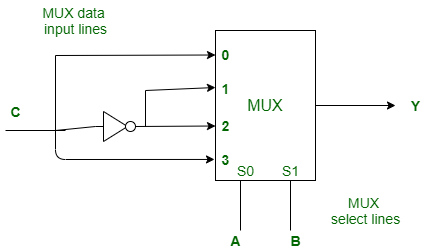GFG App
Open AppBrowser
Continue

# ISRO | ISRO CS 2020 | Question 9

Following Multiplexer circuit is equivalent to(A) Sum equation of full adder
(B) Carry equation of full adder
(C) Borrow equation for full subtractor
(D) Difference equation of a full subtractor

Explanation: According to given Multiplexer,

```Y = A′B′C+A′BC′+AB′C′+ABC
Y = A⊕B⊕C
Y = Σm(1, 2, 4, 7)```

Which is equivalent to sum equation of full adder and also difference equation of a full subtractor.

Both option (A) and (D) are correct.

Quiz of this Question

My Personal Notes arrow_drop_up